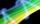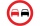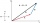Vector - 9th grade (14y) - examples

1. Find the 10Find the value of t if 2tx+5y-6=0 and 5x-4y+8=0 are perpendicular, parallel, what angle does each of the lines make with the x-axis, find the angle between the lines?
2. VectorCalculate length of the vector v⃗ = (9.75, 6.75, -6.5, -3.75, 2).
3. LineLine p passing through A[-10, 6] and has direction vector v=(3, 2). Is point B[7, 30] on the line p?
4. VectorsVector a has coordinates (8; 10) and vector b has coordinates (0; 17). If the vector c = b - a, what is the magnitude of the vector c?
5. 3d vector componentThe vector u = (3.9, u3) and the length of the vector u is 12. What is is u3?
6. VectorsFor vector w is true: w = 2u-5v. Determine coordinates of vector w if u=(3, -1), v=(12, -10)
7. Vector - basic operationsThere are given points A [-9; -2] B [2; 16] C [16; -2] and D [12; 18] a. Determine the coordinates of the vectors u=AB v=CD s=DB b. Calculate the sum of the vectors u + v c. Calculate difference of vectors u-v d. Determine the coordinates of the vector w.
8. ForcesForces with magnitudes F1 = 42N and F2 = 35N act at a common point and make an angle of 77°12'. How big is their resultant?
9. Forces on earth directionsA force of 60 N [North] and 80 N [East] is exerted on an object wigth 10 kg. What is the acceleration of the object?
10. Two forcesThe two forces F1 = 580N and F2 = 630N have the angle of 59 degrees. Calculate their resultant force F.
11. OvertakingOn the direct road, the passenger car overtakes the slower bus by starting to overtake 20 meters from the bus and after passing it ahead of it again 20 meters away. The car overtakes at a steady speed of 72 km/h, the bus goes at a steady speed of 54 km/h..
12. ParatrooperAfter the parachute is opened, the paratrooper drops to the ground at a constant speed of 2 m/s, with the sidewinding at a steady speed of 1.5 m/s. Find: a) the magnitude of its resulting velocity with respect to the ground, b) the distance of his land fr
13. Coordinates of vectorDetermine the coordinate of a vector u=CD if C(19;-7) and D(-16;-5)

We apologize, but in this category are not a lot of examples.
Do you have an interesting mathematical example that you can't solve it? Enter it, and we can try to solve it.

To this e-mail address, we will reply solution; solved examples are also published here. Please enter e-mail correctly and check whether you don't have a full mailbox.

Two vectors given by its magnitudes and by included angle can be added by our vector sum calculator.